# Polynomials

##### Class 9th Mathematics Part I MHB Solution
Practice Set 3.1
1. State whether the given algebraic expressions are polynomials? Justify. i. y + 1/y ii.…
2. Write the coefficient of in each of the given polynomial. i. m^3 ii. -3/2 + m - root…
3. Write the polynomial in x using the given information. i. Monomial with degree 7 ii.…
4. Write the degree of the given polynomials. i. root 5 ii. x^0 iii. x^2 iv. root 2m^10 -…
5. Classify the following polynomials as linear, quadratic and cubic polynomial. i. 2x^2 +…
6. Write the following polynomials in standard form. i. m^3 + 3 + 5m ii. -7y+y^5 + 3y^3 -…
7. Write the following polynomials in coefficient form. i. x^3 - 2 ii. 5y iii. 2m^4 - 3m^2…
8. Write the polynomials in index form. i. (1, 2, 3) ii. (5, 0, 0, 0, -1) iii. (-2, 2, -2,…
9. Write the appropriate polynomials in the boxes.
###### Practice Set 3.1
Question 1.

State whether the given algebraic expressions are polynomials? Justify.

i. y + 1/y

ii. 2-5√x

iii. x2 + 7x + 9

iv. 2m-2 + 7m – 5

v. 10

(i) y + 1/y is not a polynomial because in this given equation the second term becomes 1 × y-1 where -1 is not a whole number. So the given algebraic expression is not a polynomial.

(ii) 2-5√x is not a polynomial because again the power of x is 1/2 which is not a whole number.

(iii) The given algebraic expression is a polynomial in one variable because all the powers of x are whole numbers.

(iv) This expression is not polynomial because the power of the first term is -2 which is not a whole number.

(v) The number 10 is a polynomial because 10 can be represented as follows: 10 × x0 which is equal to 10. So it is a polynomial.

Question 2.

Write the coefficient of in each of the given polynomial.

i. m3

ii.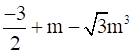iii.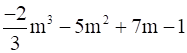i. The coefficient of any variable is the constant with which the variable is multiplied with. So the coefficient of m3 is one.

ii. The coefficient of m0 = -3/2

The coefficient of m = 1

The coefficient of m3 = -√3

iii. The coefficient of m0 = -1

The coefficient of m1 = 7

The coefficient of m2 = -5

The coefficient of m3 = -2/3

Question 3.

Write the polynomial in x using the given information.

i. Monomial with degree 7

ii. Binomial with degree 35

iii. Trinomial with degree 8

i. A polynomial is said to be monomial if it contains only one term in its entire expression. So the polynomial is as follows:

7x7

ii. A polynomial is said to be binomial if it contains only two term in its entire expression. So the polynomial is as follows:

2x35 + 7

iii. A polynomial is said to be trinomial if it contains only three term in its entire expression. So the polynomial is as follows:

2x8 + 7x + 3

Question 4.

Write the degree of the given polynomials.

i.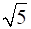ii. x0

iii. x2

iv.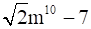v.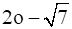vi. 7y – y3 + y5

vii. xyz + xy

viii. m3n7 – 3m5n + mn

i. The degree of a polynomial is the highest degree of its monomials (individual terms) with non-zero coefficients. This algebraic expression has zero degree.

ii. The degree of the given polynomial is zero.

iii. The degree of the given polynomial is two.

iv. The degree of the given polynomial is ten.

v. The degree of the given polynomial is one.

vi. The degree of the given polynomial is five.

vii. The degree of the given polynomial is three.

viii. The degree of the given polynomial is ten.

Question 5.

Classify the following polynomials as linear, quadratic and cubic polynomial.

i. 2x2 + 3x +1

ii. 5p

iii.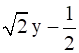iv.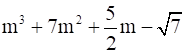v. a2

vi. 3r3

i. A polynomial is said to be quadratic if the highest power of the variable in the polynomial is two.

ii. The given polynomial is linear as the highest power of the variable is one.

iii. This polynomial is a linear one as the variable has the highest degree as one.

iv. This polynomial is a cubic polynomial because the highest power of the variable is three.

v. A polynomial is said to be quadratic if the highest power of the variable in the polynomial is two.

vi. This polynomial is a cubic polynomial because the highest power of the variable is three.

Question 6.

Write the following polynomials in standard form.

i. m3 + 3 + 5m

ii.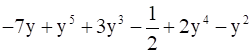i. In the standard form of any polynomial it is necessary for the power of the variable to go in descending order for each term.

So the standard form of this polynomial is as follows:

m3 + 5m + 3

ii. In the standard form of any polynomial it is necessary for the power of the variable to go in descending order for each term that is first term should consist of variable with highest power, second term should contain the variable with second highest power and so on.

So the standard form of this polynomial is as follows:

y5 + 2y4 + 3y3 –y2 -7y – 1/2

Question 7.

Write the following polynomials in coefficient form.

i. x3 – 2

ii. 5y

iii. 2m4 – 3m2 + 7

iv.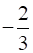i. In the coefficient form of the polynomial, the coefficient of each term of the variable present or absent is written inside the simple brackets. So the coefficient form is as follows:

⇒ x3 -2 = x3 + 0x2 + 0x – 2

∴ The given polynomial in coefficient form is:

(1, 0, 0, -2)

ii. In the coefficient form of the polynomial, the coefficient of each term of the variable present or absent is written inside the simple brackets. So the coefficient form is as follows:

⇒ 5y = 5.y + 0

Therefore, the given polynomial in coefficient form is:

(5, 0)

iii. In the coefficient form of the polynomial, the coefficient of each term of the variable present or absent is written inside the simple brackets. So, the coefficient form is as follows

2m4 – 3m2 + 7 = 2.m4 + 0.m3 – 3.m2 + 0.m + 7

Therefore, the given polynomial in coefficient form is:

(2, 0, -3, 0, 7)

iv. In the coefficient form of the polynomial, the coefficient of each term of the variable present or absent is written inside the simple brackets.

Now in this case all the powers of the variable are zero and only the constant is present.

So the coefficient form is as follows:

(-2/3)

Question 8.

Write the polynomials in index form.

i. (1, 2, 3)

ii. (5, 0, 0, 0, -1)

iii. (-2, 2, -2, 2)

i. The given representation is the coefficient form of the polynomial. So, the first coefficient denotes the highest power of the variable.

Writing in the index form, the polynomial is:

⇒ 1.x3 + 2x2 + 0.x + 3

⇒ x3 + 2x2 + 3

∴ Index form of polynomial is x3 + 2x2 + 3

ii. The given representation is the coefficient form of the polynomial. So the first coefficient denotes the highest power of the variable and the power of last term is always zero.

= 5.x4 +0.x3 + 0.x2 + 0.x – 1

= 5x4 – 1

Therefore, the index form of the polynomial is = 5x4 – 1

iii. The given representation is the coefficient form of the polynomial. So the first coefficient denotes the highest power of the variable and the power of last term is always zero.

= -2.x3 + 2.x2 – 2.x + 2

= -2x3 + 2x2 – 2x + 2

Therefore, the index form of the polynomial is = -2x3 + 2x2 – 2x + 2

Question 9.

Write the appropriate polynomials in the boxes.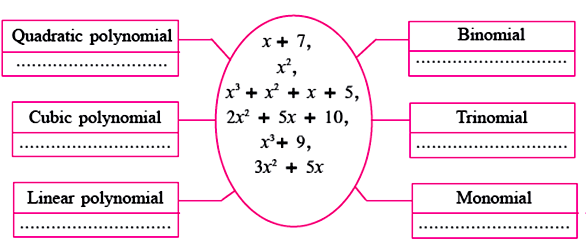A polynomial is said to be quadratic if the highest power of the variable in the polynomial is two.

The polynomial is said to be linear if the variable has the highest degree as one.

The polynomial is said to be cubic if the variable has the highest degree as three.

A polynomial is said to be monomial if it contains only one term in its entire expression.

A polynomial is said to be binomial if it contains only two terms in its entire expression.

A polynomial is said to be trinomial if it contains only three terms in its entire expression.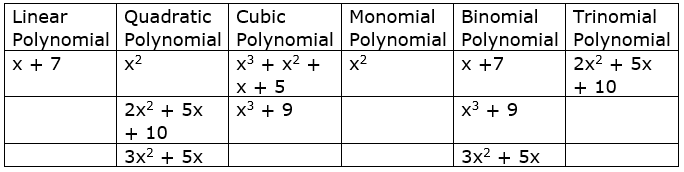## PDF FILE TO YOUR EMAIL IMMEDIATELY PURCHASE NOTES & PAPER SOLUTION. @ Rs. 50/- each (GST extra)

SUBJECTS

HINDI ENTIRE PAPER SOLUTION

MARATHI PAPER SOLUTION
SSC MATHS I PAPER SOLUTION
SSC MATHS II PAPER SOLUTION
SSC SCIENCE I PAPER SOLUTION
SSC SCIENCE II PAPER SOLUTION
SSC ENGLISH PAPER SOLUTION
SSC & HSC ENGLISH WRITING SKILL
HSC ACCOUNTS NOTES
HSC OCM NOTES
HSC ECONOMICS NOTES
HSC SECRETARIAL PRACTICE NOTES

2019 Board Paper Solution

HSC ENGLISH SET A 2019 21st February, 2019

HSC ENGLISH SET B 2019 21st February, 2019

HSC ENGLISH SET C 2019 21st February, 2019

HSC ENGLISH SET D 2019 21st February, 2019

SECRETARIAL PRACTICE (S.P) 2019 25th February, 2019

HSC XII PHYSICS 2019 25th February, 2019

CHEMISTRY XII HSC SOLUTION 27th, February, 2019

OCM PAPER SOLUTION 2019 27th, February, 2019

HSC MATHS PAPER SOLUTION COMMERCE, 2nd March, 2019

HSC MATHS PAPER SOLUTION SCIENCE 2nd, March, 2019

SSC ENGLISH STD 10 5TH MARCH, 2019.

HSC XII ACCOUNTS 2019 6th March, 2019

HSC XII BIOLOGY 2019 6TH March, 2019

HSC XII ECONOMICS 9Th March 2019

SSC Maths I March 2019 Solution 10th Standard11th, March, 2019

SSC MATHS II MARCH 2019 SOLUTION 10TH STD.13th March, 2019

SSC SCIENCE I MARCH 2019 SOLUTION 10TH STD. 15th March, 2019.

SSC SCIENCE II MARCH 2019 SOLUTION 10TH STD. 18th March, 2019.

SSC SOCIAL SCIENCE I MARCH 2019 SOLUTION20th March, 2019

SSC SOCIAL SCIENCE II MARCH 2019 SOLUTION, 22nd March, 2019

XII CBSE - BOARD - MARCH - 2019 ENGLISH - QP + SOLUTIONS, 2nd March, 2019

HSC Maharashtra Board Papers 2020

(Std 12th English Medium)

HSC ECONOMICS MARCH 2020

HSC OCM MARCH 2020

HSC ACCOUNTS MARCH 2020

HSC S.P. MARCH 2020

HSC ENGLISH MARCH 2020

HSC HINDI MARCH 2020

HSC MARATHI MARCH 2020

HSC MATHS MARCH 2020

SSC Maharashtra Board Papers 2020

(Std 10th English Medium)

English MARCH 2020

HindI MARCH 2020

Hindi (Composite) MARCH 2020

Marathi MARCH 2020

Mathematics (Paper 1) MARCH 2020

Mathematics (Paper 2) MARCH 2020

Sanskrit MARCH 2020

Important-formula

THANKS